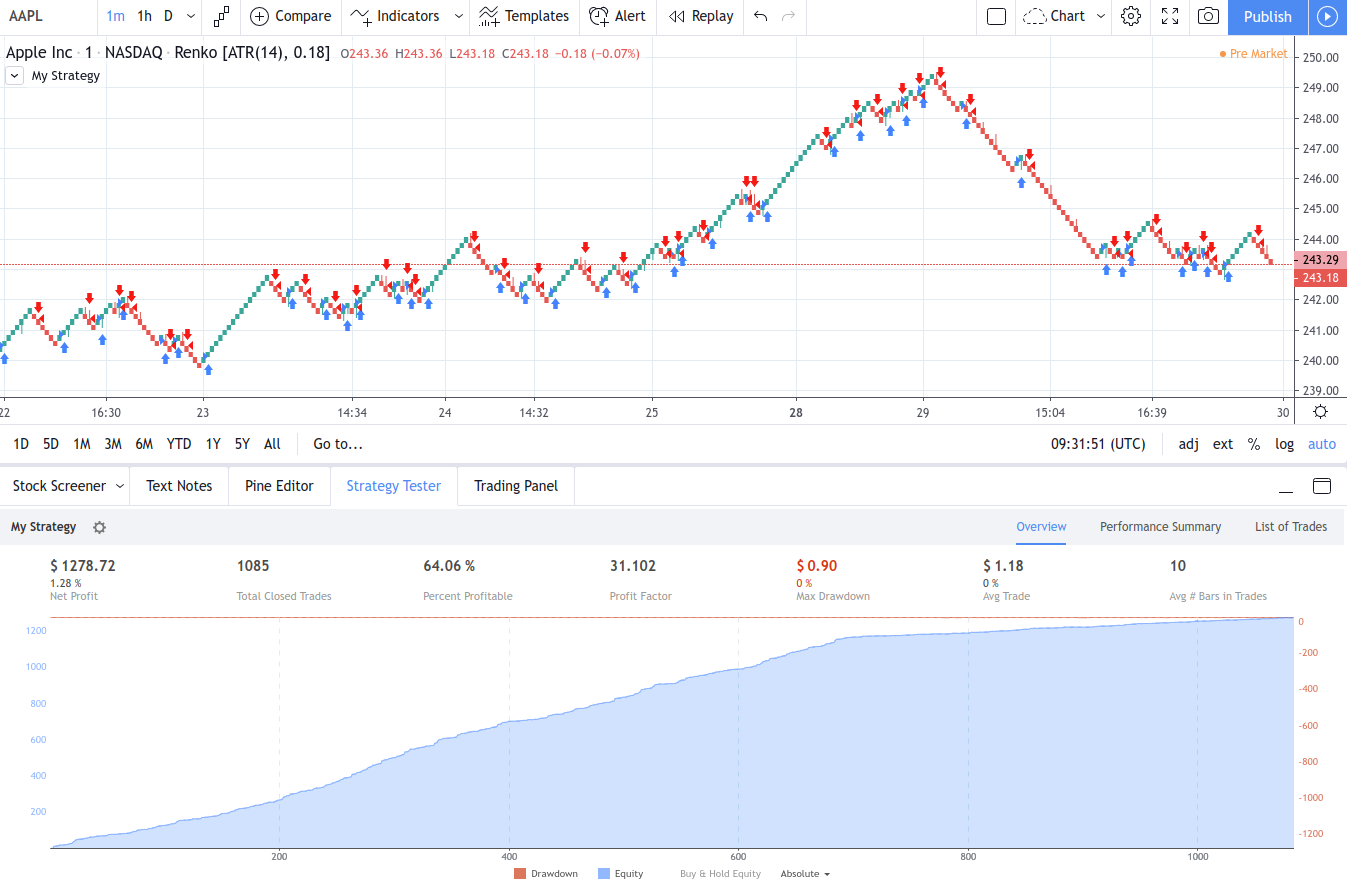# 策略在非標準圖表類型上計算錯誤(平均K線、磚形圖等)

``//@version=4 strategy("My Strategy", overlay=true) longCondition = open<close if (longCondition) strategy.entry("My Long Entry Id", strategy.long) shortCondition = open>close if (shortCondition) strategy.entry("My Short Entry Id", strategy.short)//@version=4 strategy("My Strategy", overlay=true) longCondition = open<close if (longCondition) strategy.entry("My Long Entry Id", strategy.long) shortCondition = open>close if (shortCondition) strategy.entry("My Short Entry Id", strategy.short)//@version=4 strategy("My Strategy", overlay=true) longCondition = open<close if (longCondition) strategy.entry("My Long Entry Id", strategy.long) shortCondition = open>close if (shortCondition) strategy.entry("My Short Entry Id", strategy.short)//@version=4 strategy("My Strategy", overlay=true) longCondition = open<close if (longCondition) strategy.entry("My Long Entry Id", strategy.long) shortCondition = open>close if (shortCondition) strategy.entry("My Short Entry Id", strategy.short)//@version=4 strategy("My Strategy", overlay=true) longCondition = open<close if (longCondition) strategy.entry("My Long Entry Id", strategy.long) shortCondition = open>close if (shortCondition) strategy.entry("My Short Entry Id", strategy.short)//@version=4 strategy("My Strategy", overlay=true) longCondition = open<close if (longCondition) strategy.entry("My Long Entry Id", strategy.long) shortCondition = open>close if (shortCondition) strategy.entry("My Short Entry Id", strategy.short)``
HTML